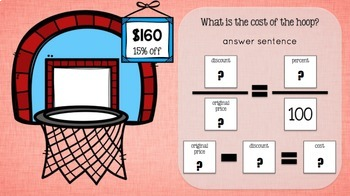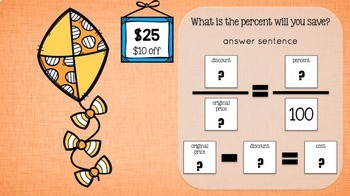# Ratio and Percents: What Does it Cost? for the Google ClassroomSubject
Resource Type
File Type
PDF (33 MB|22 pages)
Standards
\$2.40
List Price:
\$3.00
You Save:
\$0.60
\$2.40
List Price:
\$3.00
You Save:
\$0.60
• Product Description
• Standards

Works well for Distance Learning! Use ratios to solve real world math problems with this digital Google Slides resource which contains digital task cards. Students find the discounted price of an item given the original price and a percent off. Students also find the percentage saved when purchasing an item at a discounted price. Connect ratios and percents to real life by solving problems that involve shopping.

Standards:

6.RP.A.3

Use ratio and rate reasoning to solve real world and mathematical problems.

6.RP.A.3.C

Find a percent of a quantity of a rate per 100. Solve problems involving finding the whole given a part and the percent.

Find a percent of a quantity as a rate per 100 (e.g., 30% of a quantity means 30/100 times the quantity); solve problems involving finding the whole, given a part and the percent.
Use ratio and rate reasoning to solve real-world and mathematical problems, e.g., by reasoning about tables of equivalent ratios, tape diagrams, double number line diagrams, or equations.
Total Pages
22 pages